# Defining electrodes as neighbours for cluster-level statistics

The following code demonstrates how to make a layout for the 21 channels of the original 10-20 system.

Important to realize is that EEG caps and electrode arrangements have subtle variations between labs, even if you use a so-called standard layout. e.g., your particular selection of 64-channels from the extended 10-20 (or 10-10, or 10%) system can contain another subset than that used in another lab.

Although FieldTrip includes some functions that help you to construct a neighbour definition, you are yourself responsible to ensure it matches your measurement setup. The definition of neighbours influences how clusters will be formed: channels with many neighbours are be more likely to be part of large clusters than channels with few neighbours. As such the neighbour definition influences the clustering process and the statistical sensitivity. The false-alarm rate is not affected, as explained in the Nonparametric statistical testing of EEG- and MEG-data paper.

## Neighbours based on 2D layouts

It first starts off with the 2D layout of these channels. This 2D layout is basically a flat pancake projection of the 3D positions. As with any cartographic projection, this will cause geometrical distortions, especially along the edes of the sphere.

``````[ftver, ftpath] = ft_version;
cd(fullfile(ftpath, 'template', 'layout'));

cfg = [];
cfg.layout = 'elec1020.lay';
cfg.feedback = 'yes';
layout = ft_prepare_layout(cfg);
``````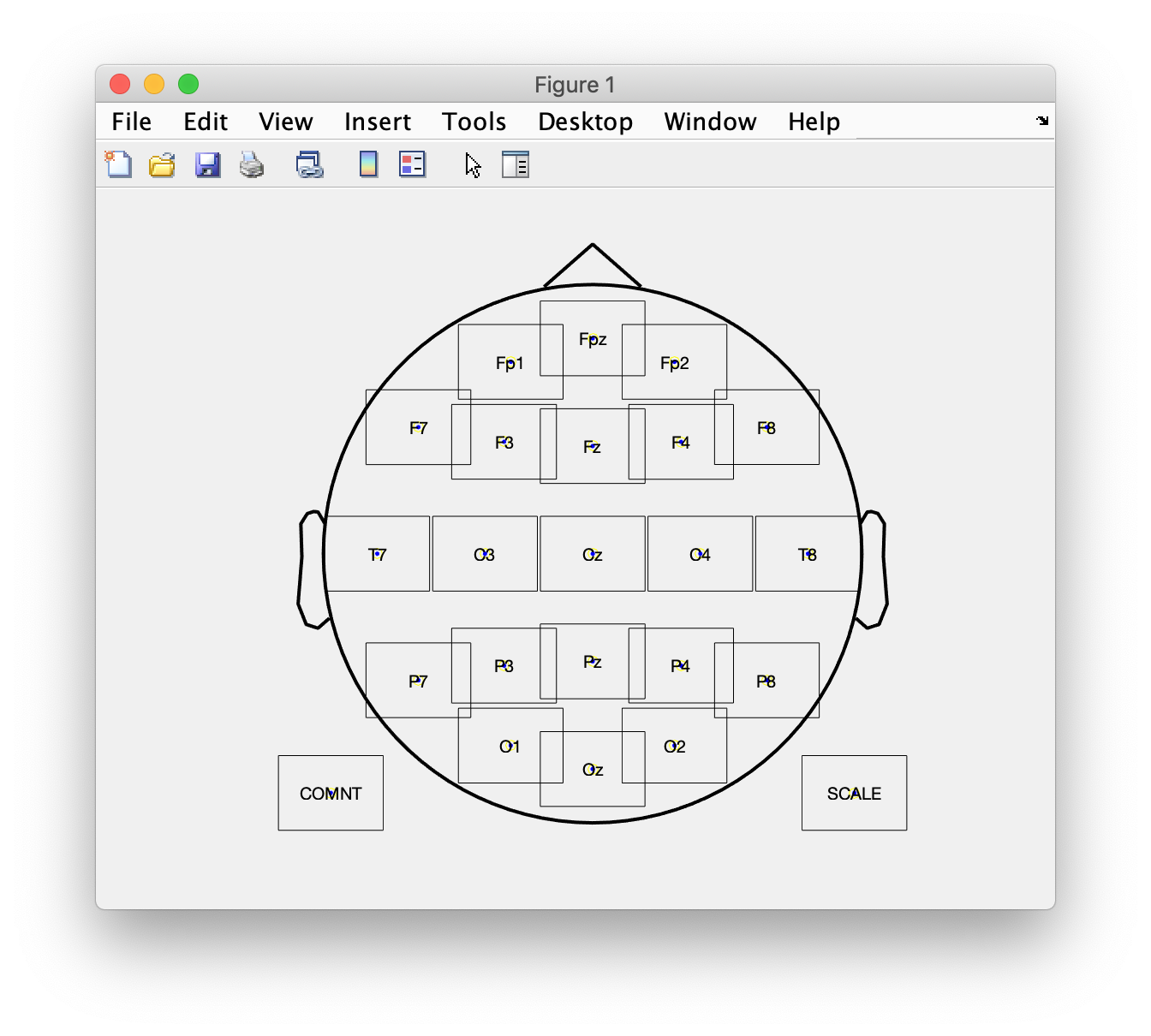With ft_prepare_neighbours is possible to use a triangulation to find the neighbours. The initial triangulation is rather sparse, therefore the default is to also compress it along the horizontal and vertical and combine the three triangulations for determining the neighbours. This results in edges that cross each other.

``````cfg = [];
cfg.layout = layout;
cfg.method = 'triangulation';
cfg.compress = 'yes';
cfg.feedback = 'yes';
neighbours1 = ft_prepare_neighbours(cfg);
``````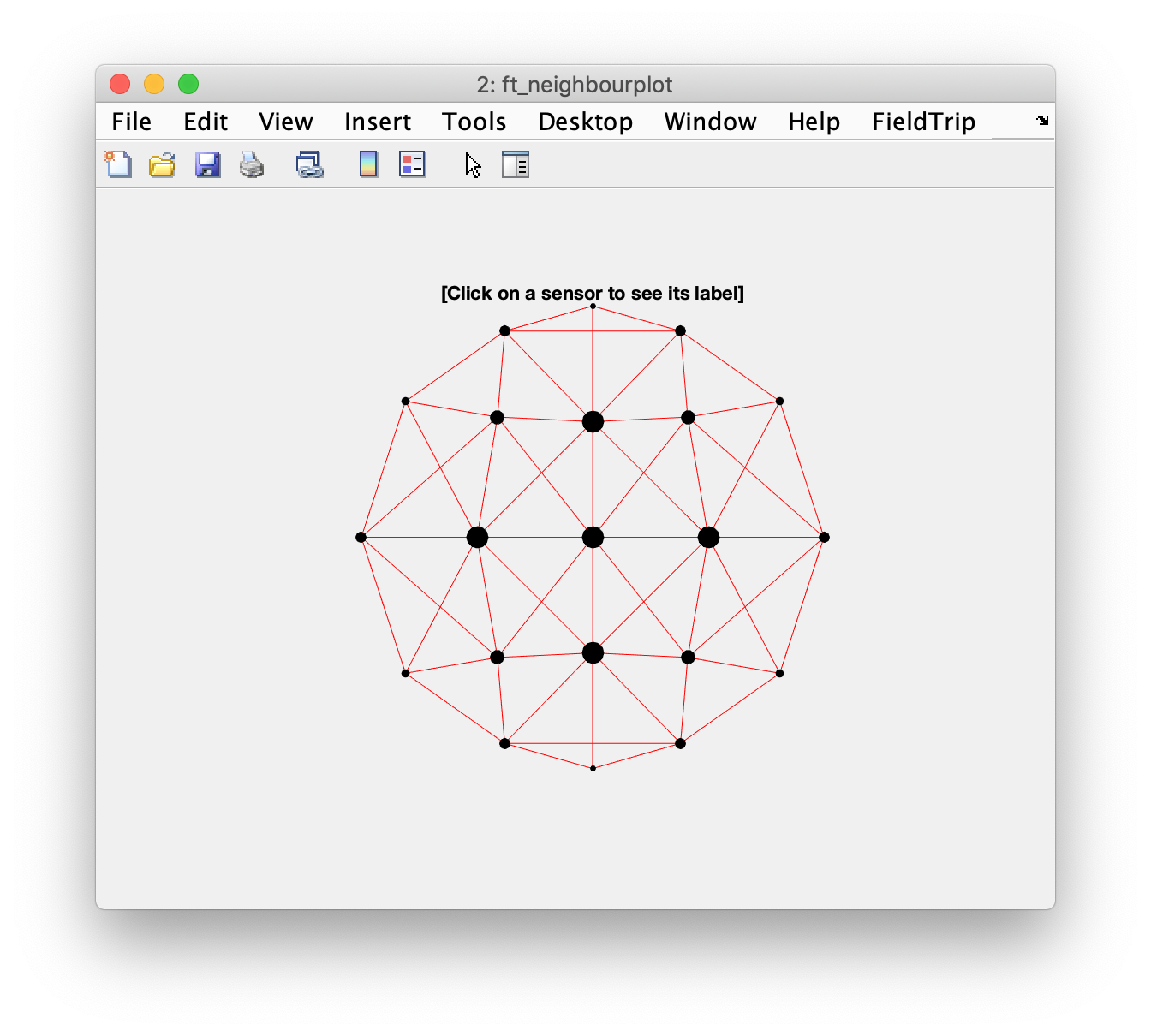Without compressing we can get the standard Delaunay triangulation, which is more sparse and does not have any crossings.

``````cfg = [];
cfg.layout = layout;
cfg.method = 'triangulation';
cfg.compress = 'no';
cfg.feedback = 'yes';
neighbours2 = ft_prepare_neighbours(cfg);
``````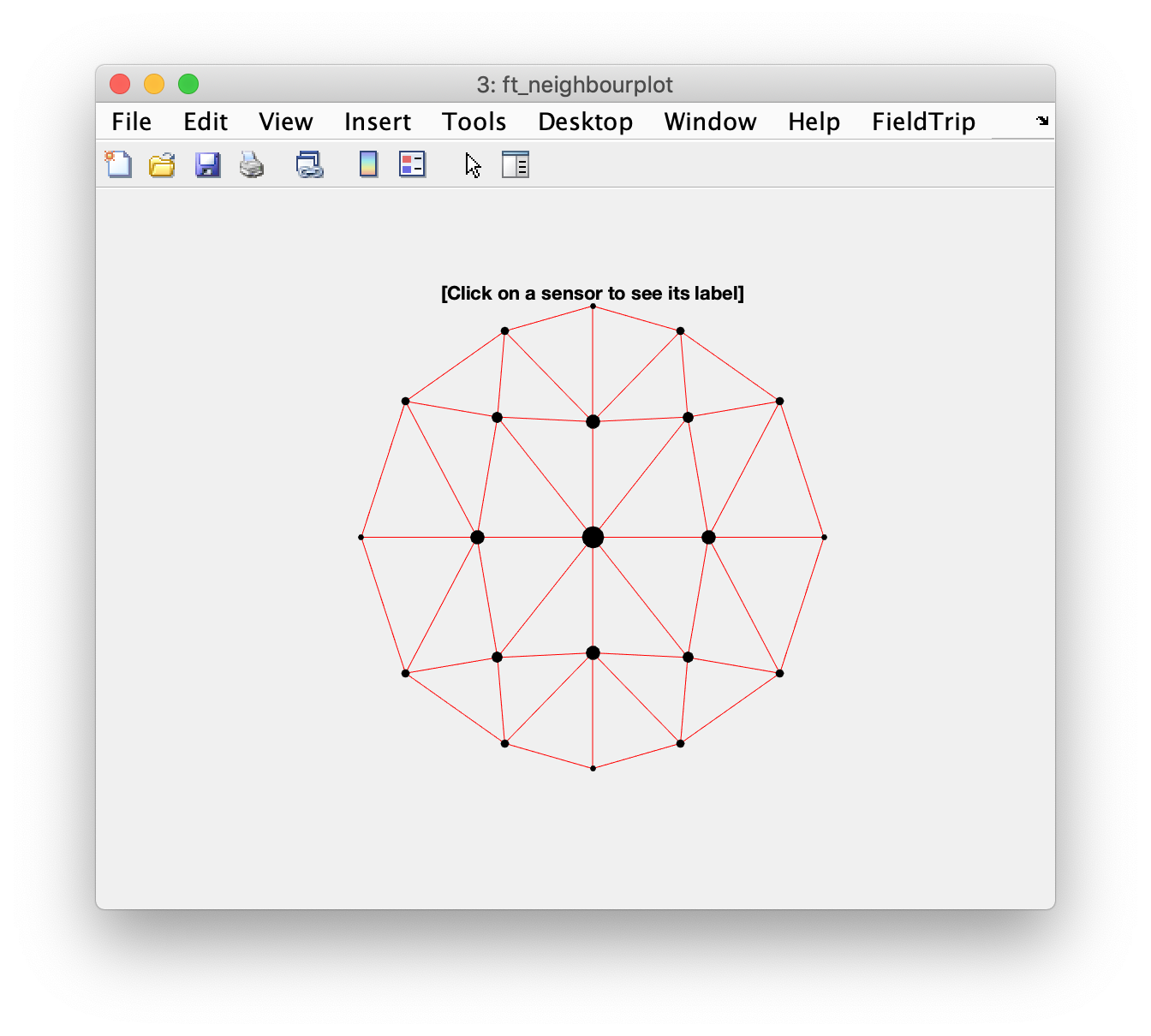We can also use the distance between electrodes to decide on the neighbours. This requires playing with the value for the `cfg.neighbourdist` parameter.

``````cfg = [];
cfg.layout = layout;
cfg.method = 'distance';
cfg.feedback = 'yes';
cfg.neighbourdist = 0.30;
neighbours3 = ft_prepare_neighbours(cfg);
``````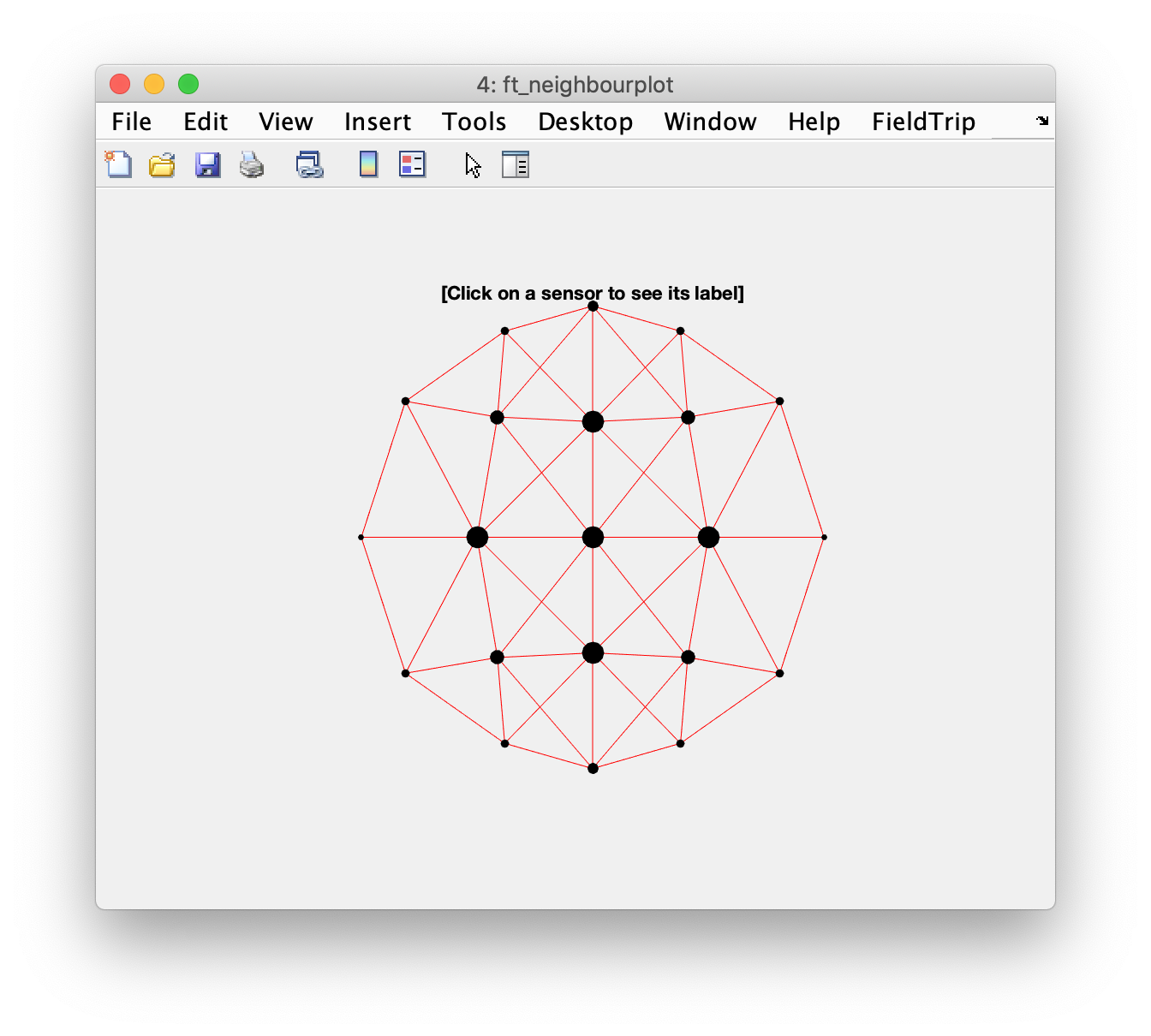A smaller distance will result in fewer neighbours.

``````cfg = [];
cfg.layout = layout;
cfg.method = 'distance';
cfg.feedback = 'yes';
cfg.neighbourdist = 0.25;
neighbours4 = ft_prepare_neighbours(cfg);
``````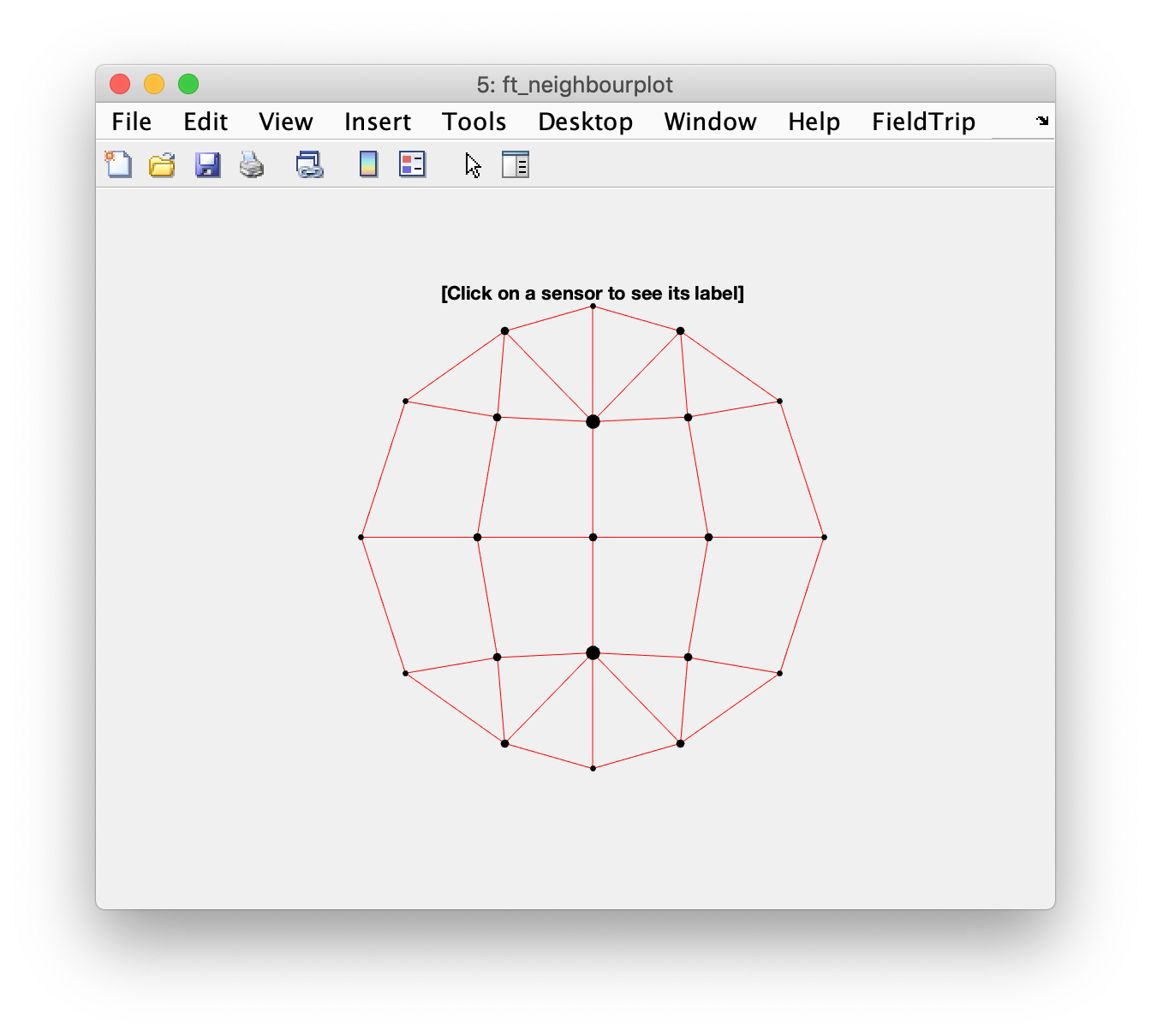## Neighbours based on 3D positions

Rather than using the flattened 2D projection of the electrodes in the layout, we can also start off with a 3D representation of the positions. In the `template/electrode` directory you can find template positions for various EEG caps. The `standard_1020` not only includes the 21 original positions, but contains the positions of the extended 10% system. This also includes the mastoids (M1, M2), earlobes (A1, A2) and the electrode positions T3, T4, T5 and T6, which are simply different names for T7, T8, P7 and P8.

Having two electrodes on exactly the same place (like T3 and T7) confuses the code that determines the orientation of the electrode that is used in plotting, not all orientations are therefore correct.

``````[ftver, ftpath] = ft_version;
cd(fullfile(ftpath, 'template', 'electrode'));

% there are some of which the orientation cannot be determined (T3, T4, T5, T6)
ft_plot_sens(elec, 'label', 'yes', 'elecshape', 'disc', 'elecsize', 10, 'facecolor', [0.8 0.8 1.0])
% give it a stronger 3D appearance
``````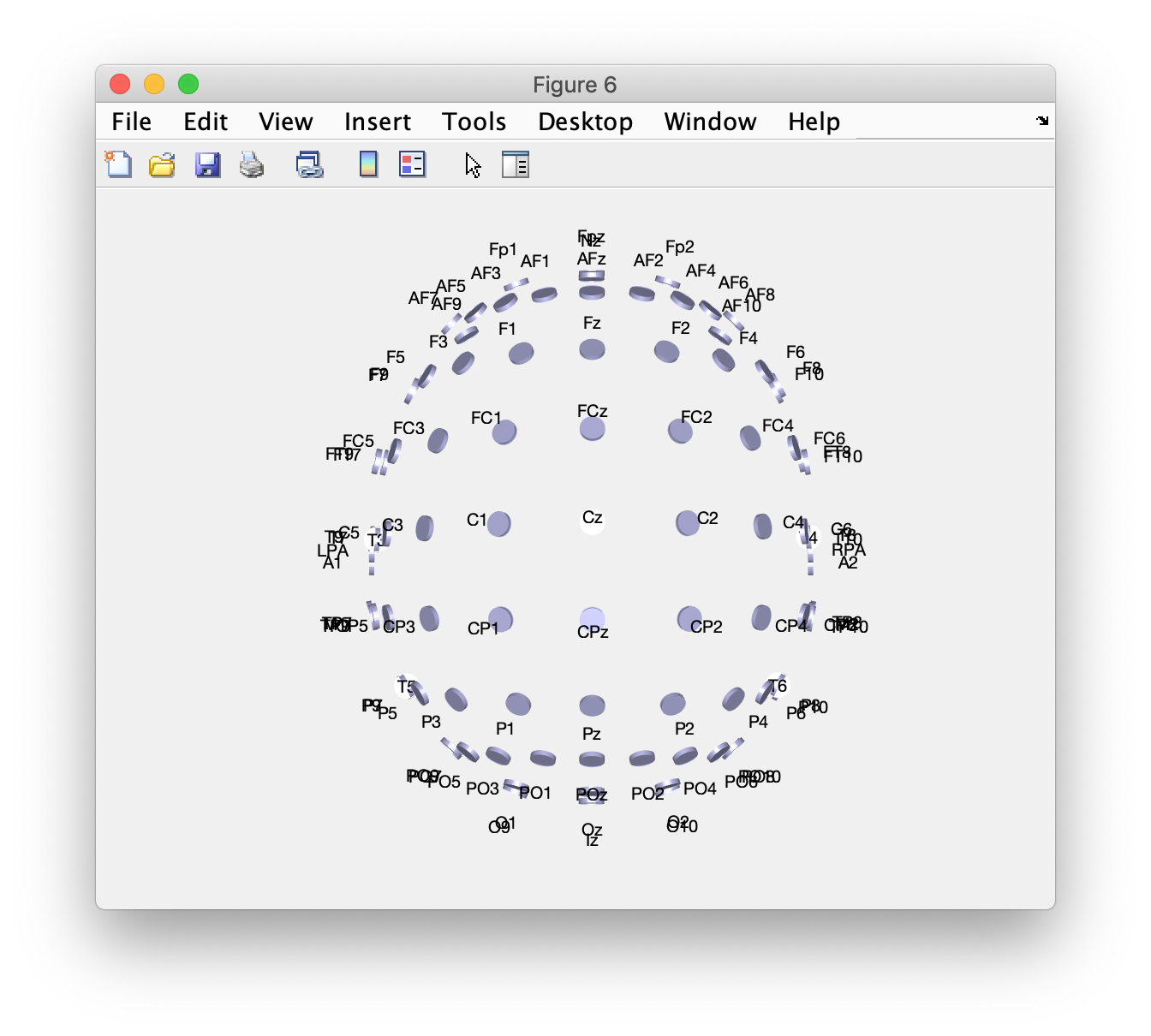We can again use the triangulation method. This temporarily projects the electrodes on a 2D plane and uses the Delaunay method, including crossing connections.

``````cfg = [];
cfg.elec = elec;
cfg.channel = {'Fp1', 'Fpz', 'Fp2', 'F7', 'F3', 'Fz', 'F4', 'F8', 'T7', 'C3', 'Cz', 'C4', 'T8', 'P7', 'P3', 'Pz', 'P4', 'P8', 'O1', 'Oz', 'O2'};
cfg.method = 'triangulation';
cfg.compress = 'yes';
cfg.feedback = 'yes';
neighbours5 = ft_prepare_neighbours(cfg);
``````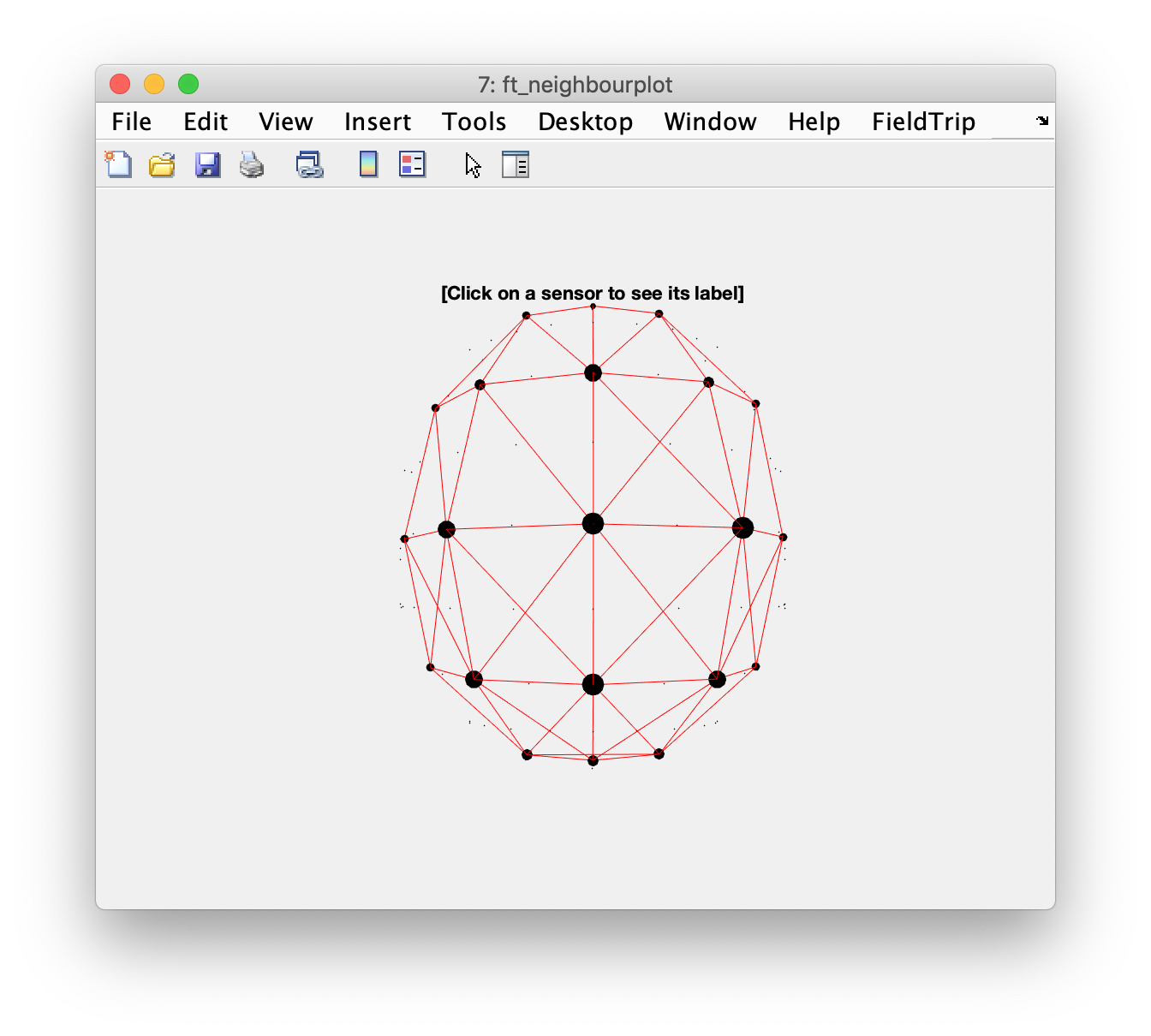This is the Delaunay method without crossing connections.

``````cfg = [];
cfg.elec = elec;
cfg.channel = {'Fp1', 'Fpz', 'Fp2', 'F7', 'F3', 'Fz', 'F4', 'F8', 'T7', 'C3', 'Cz', 'C4', 'T8', 'P7', 'P3', 'Pz', 'P4', 'P8', 'O1', 'Oz', 'O2'};
cfg.method = 'triangulation';
cfg.compress = 'no';
cfg.feedback = 'yes';
neighbours6 = ft_prepare_neighbours(cfg);
``````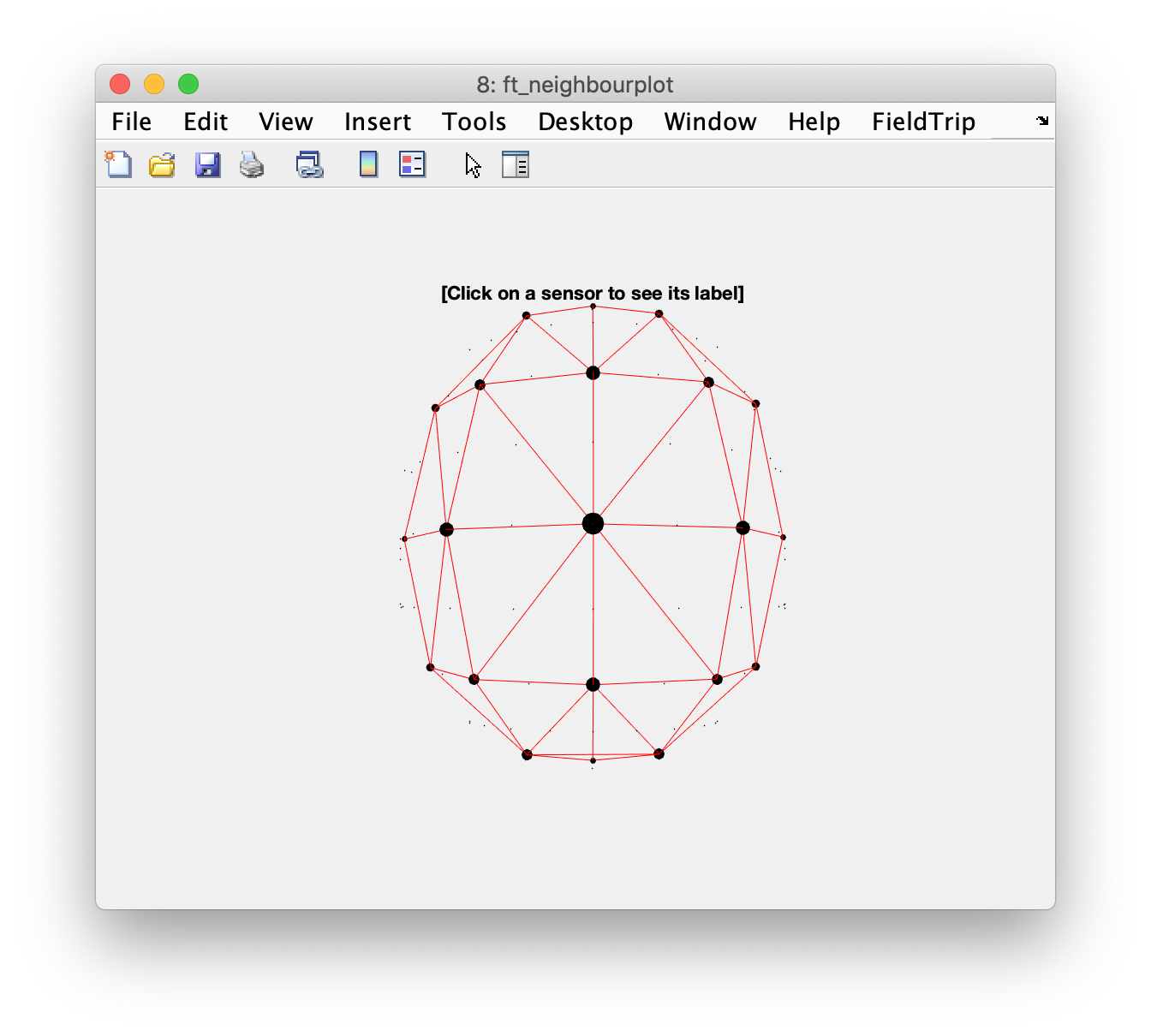Since we now have 3D positions, the distance-based method is now easier to interpret, as there are no distortions along the edges.

``````cfg = [];
cfg.elec = elec;
cfg.channel = {'Fp1', 'Fpz', 'Fp2', 'F7', 'F3', 'Fz', 'F4', 'F8', 'T7', 'C3', 'Cz', 'C4', 'T8', 'P7', 'P3', 'Pz', 'P4', 'P8', 'O1', 'Oz', 'O2'};
cfg.method = 'distance';
cfg.feedback = 'yes';
cfg.neighbourdist = 100;
neighbours7 = ft_prepare_neighbours(cfg);
``````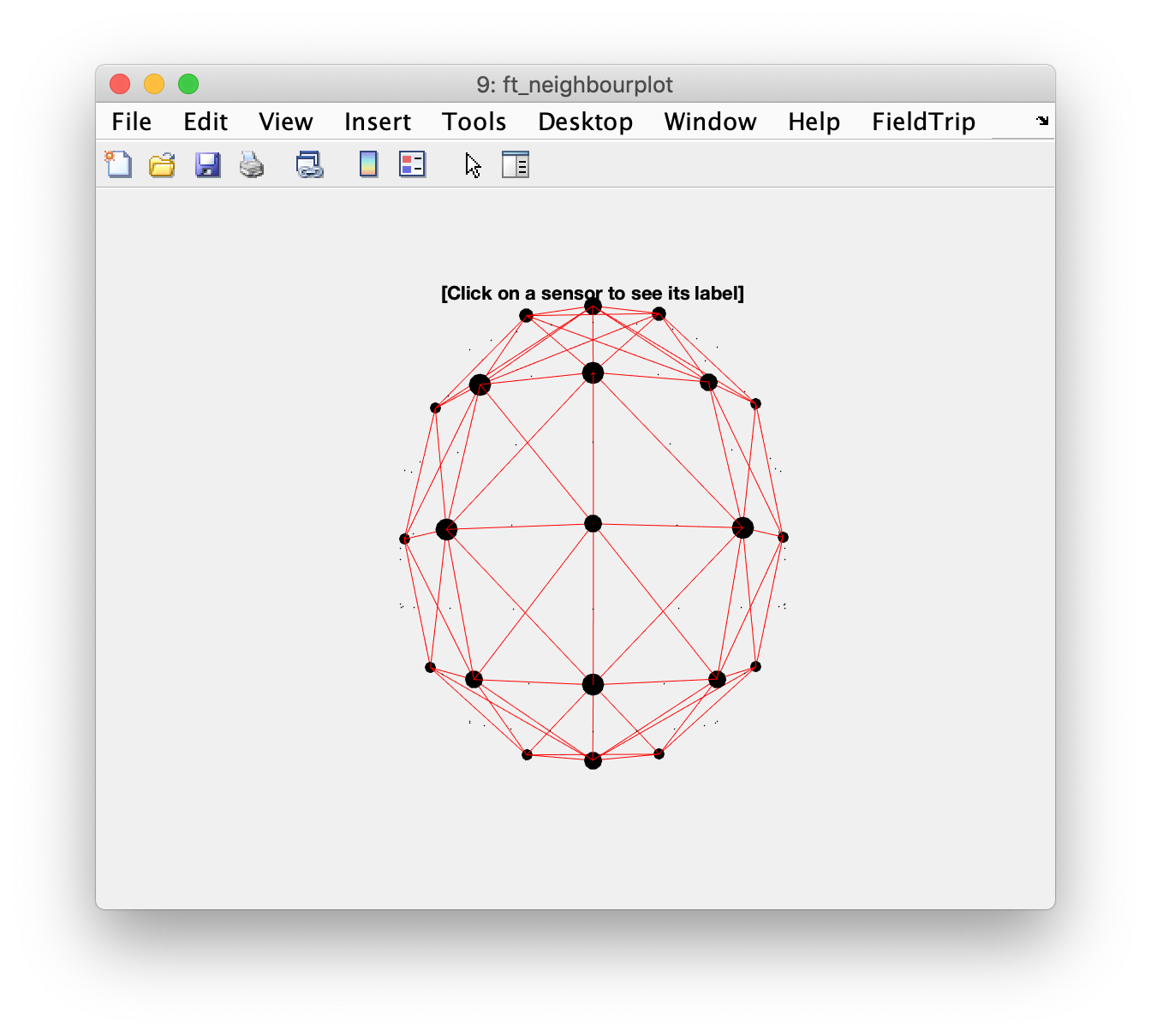A slightly smaller distance can have a large effect of which electrodes are detected as neighbours.

``````cfg = [];
cfg.elec = elec;
cfg.channel = {'Fp1', 'Fpz', 'Fp2', 'F7', 'F3', 'Fz', 'F4', 'F8', 'T7', 'C3', 'Cz', 'C4', 'T8', 'P7', 'P3', 'Pz', 'P4', 'P8', 'O1', 'Oz', 'O2'};
cfg.method = 'distance';
cfg.feedback = 'yes';
cfg.neighbourdist = 80;
neighbours8 = ft_prepare_neighbours(cfg);
``````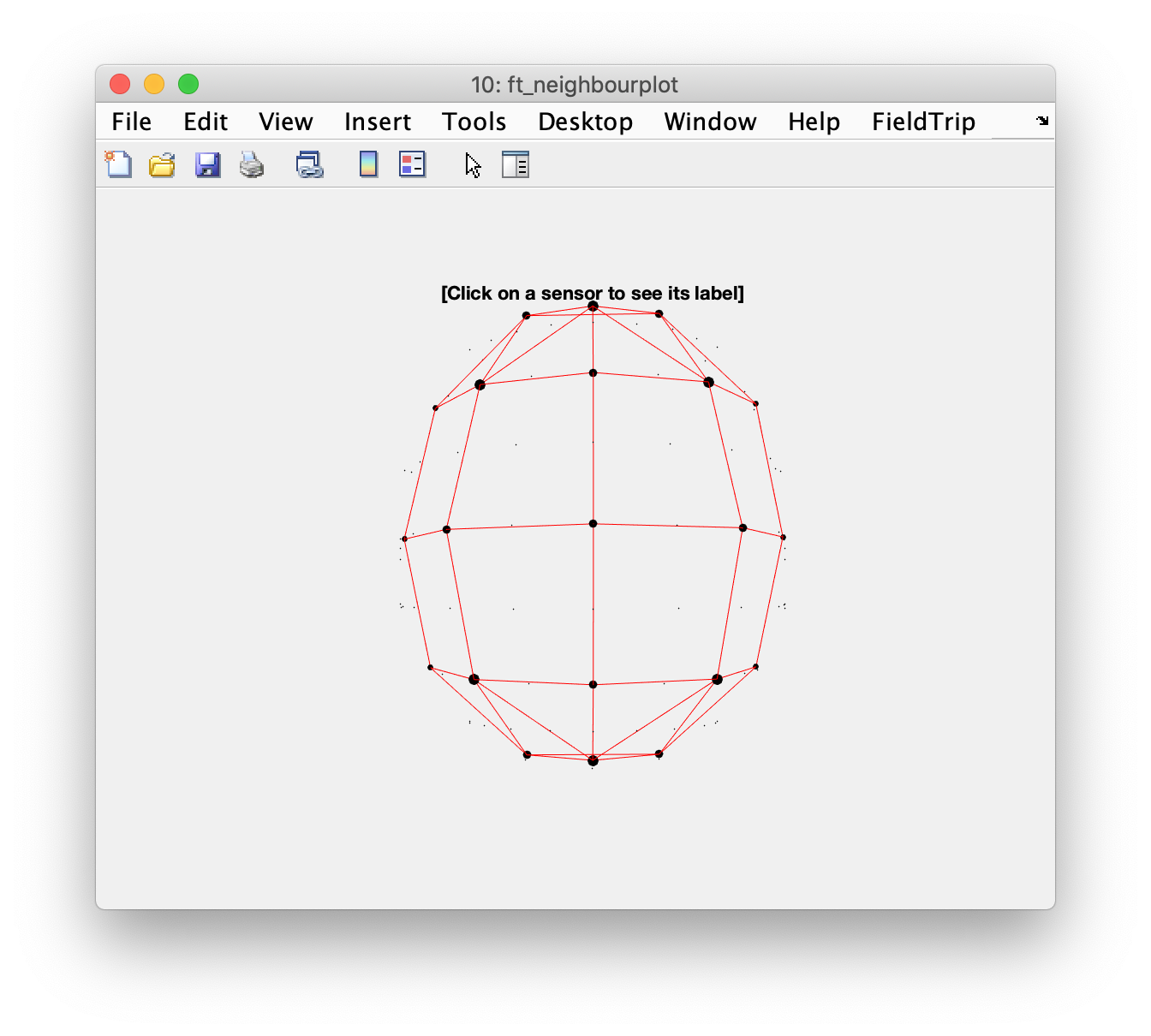If you rotate the 3D figure, you can see that O1 and O2 are considered neighbours, as well as Fp1 and Fp2. They are quite close to each other, but I would not consider them direct neighbours, since Oz and Fpz are in between. To improve on the neighbour structure, you would edit the structure that it returns.

Note that if you remove a neighbour from one channel, you probably also want to remove it the opposite way around. In general we would consider neighbours symmetric, i.e., if channel “A” is a neighbour of channel “B”, channel “B” will also be the neighbour of “A”.

You can use the printstruct function to display the structure on screen and copy-and-paste it into your script, where you can make additional refinements.

``````printstruct('neighbours', neighbours8)

neighbours(1).label = 'Fp1';
neighbours(1).neighblabel = { 'Fpz', 'Fp2', 'F7', 'F3' };
neighbours(2).label = 'Fpz';
neighbours(2).neighblabel = { 'Fp1', 'Fp2', 'F3', 'Fz', 'F4' };
neighbours(3).label = 'Fp2';
neighbours(3).neighblabel = { 'Fp1', 'Fpz', 'F4', 'F8' };
neighbours(4).label = 'F7';
neighbours(4).neighblabel = { 'Fp1', 'F3', 'T7' };
neighbours(5).label = 'F3';
neighbours(5).neighblabel = { 'Fp1', 'Fpz', 'F7', 'Fz', 'C3' };
neighbours(6).label = 'Fz';
neighbours(6).neighblabel = { 'Fpz', 'F3', 'F4', 'Cz' };
neighbours(7).label = 'F4';
neighbours(7).neighblabel = { 'Fpz', 'Fp2', 'Fz', 'F8', 'C4' };
neighbours(8).label = 'F8';
neighbours(8).neighblabel = { 'Fp2', 'F4', 'T8' };
neighbours(9).label = 'T7';
neighbours(9).neighblabel = { 'F7', 'C3', 'P7' };
neighbours(10).label = 'C3';
neighbours(10).neighblabel = { 'F3', 'T7', 'Cz', 'P3' };
neighbours(11).label = 'Cz';
neighbours(11).neighblabel = { 'Fz', 'C3', 'C4', 'Pz' };
neighbours(12).label = 'C4';
neighbours(12).neighblabel = { 'F4', 'Cz', 'T8', 'P4' };
neighbours(13).label = 'T8';
neighbours(13).neighblabel = { 'F8', 'C4', 'P8' };
neighbours(14).label = 'P7';
neighbours(14).neighblabel = { 'T7', 'P3', 'O1' };
neighbours(15).label = 'P3';
neighbours(15).neighblabel = { 'C3', 'P7', 'Pz', 'O1', 'Oz' };
neighbours(16).label = 'Pz';
neighbours(16).neighblabel = { 'Cz', 'P3', 'P4', 'Oz' };
neighbours(17).label = 'P4';
neighbours(17).neighblabel = { 'C4', 'Pz', 'P8', 'Oz', 'O2' };
neighbours(18).label = 'P8';
neighbours(18).neighblabel = { 'T8', 'P4', 'O2' };
neighbours(19).label = 'O1';
neighbours(19).neighblabel = { 'P7', 'P3', 'Oz', 'O2' };
neighbours(20).label = 'Oz';
neighbours(20).neighblabel = { 'P3', 'Pz', 'P4', 'O1', 'O2' };
neighbours(21).label = 'O2';
neighbours(21).neighblabel = { 'P4', 'P8', 'O1', 'Oz' };
``````

You can also use ft_neighbourplot, clicking on one electrode and then another allows to graphically add or remove a connection.

``````cfg = [];
cfg.elec = elec;
cfg.neighbours = neighbours;
cfg.enableedit = 'yes';
cfg.verbose = 'yes';
neighbours_edited = ft_neighbourplot(cfg);
``````